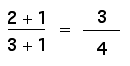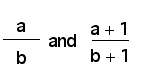# Proper Fraction Fury

Suppose you start with a proper fraction (where the numerator is less than the denominator), and you add 1 to the numerator and to the denominator. For example, if you started with 2/3, then you’d getIs this procedure of adding 1 to the numerator and the denominator the same as adding the number 1 to the original fraction? Explain your reasoning.

Try several examples (with proper fractions), comparing the sizes of the original fraction and the new fraction. What do you notice?

Give a general explanation for what you observe. In other words, compare the sizes of(Source: Insights in Arithmetic and Geometry, Sybilla Beckmann, University of Georgia, Department of Mathematics, 1998.)